# Reason + sets - math problems

#### Number of problems found: 45

• Marriage sttusIn our city, there are 3/5 of the women married to 2/3 of the men. Find what part of the population is free.
• Subsets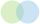How many 19 element's subsets can be made from the 26 element set?
• Dices throwsWhat is the probability that the two throws of the dice: a) Six falls even once b) Six will fall at least once
• Classroom 3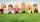How many children are in the classroom, where 13 children are higher than Lenka and 9 children lower than Lenka?
• Pizza master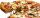Master says that he can splits pizza to 16 parts by five equals straight cuts. Is it possible?
• Pairs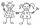From the five girls and four boys teachers have to choose one pair of boy and girl. A) How many such pairs of (M + F)? B) How many pairs where only boys (M + M)? C) How many are all possible pairs?
• Rings - intersect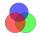There are 15 pupils on the sporting ring. 10 pupils go to football, 8 pupils go to floorball. How many pupils go to both rings at the same time?
• Trousers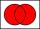In the class was 12 students. Nine students wearing trousers and turtleneck eight. How many students worn trousers with a turtleneck?
• Left handedIt is known that 25% of the population is left-handed. What is the probability that there is a maximum of three left-handers at a seminar where there are 30 participants?
• Gloves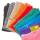Petra has ten pairs of gloves in the closet. Six pairs are blue, 4 pairs are yellow. How many pieces of gloves need to be pulled out at least when she pull them out in the dark and want to have one complete one color pair?
• Fall sum or sameFind the probability that if you roll two dice, it will fall the sum of 10, or the same number will fall on both dice.
• Language courses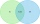Of the company's 60 employees, 28 attend an English course, 17 take a German course, and 20 do not attend any of these courses. How many employees attend both courses?
• Probability of intersectionThree students have a probability of 0.7,0.5 and 0.4 to graduated from university respectively. What is the probability that at least one of them will be graduated?
• Double probabilityThe probability of success of the planned action is 60%. What is the probability that success will be achieved at least once if this action is repeated twice?
• Buffet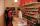In the school buffet were 30 children. 21 children bought sandwiches and 15 children bought lemonade. Four children did not buy anything. How many children bought lemonade and sandwiche?
• Skoda carsThere were 16 passenger cars in the parking. It was the 10 blue and 10 Skoda cars. How many are blue Skoda cars in the parking?
• Glasses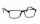There are 36 pupils in the class. Nine girls wear glasses. Boys with glasses are five less than girls without glasses. Boys without glasses are two times more than girls without glasses. How many boys and how many girls?
• PupilsThere are 27 pupils in the classroom. They can swim 21 and ski nine pupils. Neither swim nor ski three pupils. How many pupils can swim and ski?
• Brothers and sisters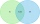There are 35 children in the class, 23 of them have a brother, and 27 of them have a sister. How many children have both a brother and a sister when there are 5 children in the class who have no brother or sister?
• Math logicThere are 20 children in the group, each two children have a different name. Alena and John are among them. How many ways can we choose 8 children to be among the selected A) was John B) was John and Alena C) at least one was Alena, John D) maximum one wa

Do you have an interesting mathematical word problem that you can't solve it? Submit a math problem, and we can try to solve it.

We will send a solution to your e-mail address. Solved examples are also published here. Please enter the e-mail correctly and check whether you don't have a full mailbox.

Please do not submit problems from current active competitions such as Mathematical Olympiad, correspondence seminars etc...

Reason - math word problems. Sets - math word problems.